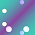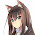## Wednesday, February 3, 2016

### From mathematics to map-reduce

There's more mathematics to programming than meets the eye. This post will highlight one such connection that explains the link between map-reduce and category theory. I will then conclude with some wild speculation about what this might imply for future programming paradigms.

This post assumes that you already know Haskell and explains the mathematics behind the map-reduce using Haskell concepts and terminology. This means that this post will oversimplify some of the category theory concepts in order to embed them in Haskell, but the overall gist will still be correct.

# Background (Isomorphism)

In Haskell, we like to say that two types, `s` and `t`, are "isomorphic" if and only if there are two functions, `fw` and `bw`, of types

``````fw :: s -> t
bw :: t -> s``````

... that are inverse of each other:

``````fw . bw = id
bw . fw = id``````

We will use the symbol `≅` to denote that two types are isomorphic. So, for example, we would summarize all of the above by just writing:

``s ≅ t``

The fully general definition of isomorphism from category theory is actually much broader than this, but this definition will do for now.

Given two functors, `f` and `g`, `f` is left-adjoint to `g` if and only if:

``f a -> b ≅ a -> g b``

In other words, for them to be adjoint there must be two functions, `fw` and `bw` of types:

``````fw :: (f a -> b) -> (a -> g b)
bw :: (a -> g b) -> (f a -> b)``````

... such that:

``````fw . bw = id
bw . fw = id``````

These "functors" are not necessarily the same as Haskell's `Functor` class. The category theory definition of "functor" is more general than Haskell's `Functor` class and we'll be taking advantage of that extra generality in the next section.

# Free functors

Imagine a functor named `g` that acted more like a type-level function that transforms one type into another type. In this case, `g` will be a function that erases a constraint named `C`. For example:

``````-- `g` is a *type-level* function, and `t` is a *type*
g (C t => t) = t``````

In other words, `g` "forgets" the `C` constraint on type `t`. We call `g` a "forgetful functor".

If some other functor, `f` is left-adjoint to `g` then we say that `f` is the "free `C`" (where `C` is the constraint that `g` "forgets").

In other words, a "free `C`" is a functor that is left-adjoint to another functor that forgets the constraint `C`.

# Free monoid

The list type constructor, `[]`, is the "free `Monoid`"

The "free `Monoid`" is, by definition, a functor `[]` that is left-adjoint to some other functor `g` that deletes `Monoid` constraints.

When we say that `g` deletes `Monoid` constraints we mean that:

``g (Monoid m => m) = m``

... and when we say that `[]` is left-adjoint to `g` that means that:

``[] a -> b ≅ a -> g b``

... and the type `[a]` is syntactic sugar for `[] a`, so we can also write:

``[a] -> b ≅ a -> g b``

Now substitute `b` with some type with a `Monoid` constraint, like this one:

``b = Monoid m => m``

That gives us:

``[a] -> (Monoid m => m) ≅ a -> g (Monoid m => m)``

... and since `g` deletes `Monoid` constraints, that leaves us with:

``[a] -> (Monoid m => m) ≅ a -> m``

The above isomorphism in turn implies that there must be two functions, `fw` and `bw`, of types:

``````fw :: ([a] -> (Monoid m => m)) -> (a -> m)
bw :: (a -> m) -> ([a] -> (Monoid m => m))``````

... and these two functions must be inverses of each other:

``````fw . bw = id
bw . fw = id``````

We can pull out the `Monoid` constraints to the left to give us these more idiomatic types:

``````fw :: (Monoid m => [a] -> m)) -> (a -> m)
bw :: Monoid m => ( a  -> m) -> ([a] -> m)``````

Both of these types have "obvious" implementations:

``````fw :: (Monoid m => [a] -> m)) -> (a -> m)
fw k x = k [x]

bw :: Monoid m => (a -> m) -> ([a] -> m)
bw k xs = mconcat (map k xs)``````

Now we need to prove that the `fw` and `bw` functions are inverse of each other. Here are the proofs:

``````-- Proof #1
fw . bw

-- eta-expand
= \k -> fw (bw k)

-- eta-expand
= \k x -> fw (bw k) x

-- Definition of `fw`
= \k x -> bw k [x]

-- Definition of `bw`
= \k x -> mconcat (map k [x])

-- Definition of `map`
= \k x -> mconcat [k x]

-- Definition of `mconcat`
= \k x -> k x

-- eta-reduce
= \k -> k

-- Definition of `id`
= id

-- Proof #2
bw . fw

-- eta-expand
= \k -> bw (fw k)

-- eta-expand
= \k xs -> bw (fw k) xs

-- Definition of `bw`
= \k xs -> mconcat (map (fw k) xs)

-- eta-expand
= \k xs -> mconcat (map (\x -> fw k x) xs)

-- Definition of `fw`
= \k xs -> mconcat (map (\x -> k [x]) xs)

-- map (f . g) = map f . map g
= \k xs -> mconcat (map k (map (\x -> [x]) xs))

-- ... and then a miracle occurs ...
--
-- In all seriousness this step uses a "free theorem" which says
-- that:
--
--     forall (k :: Monoid m => [a] -> m) . mconcat . map k = k . mconcat
--
-- We haven't covered free theorems, but you can read more about them
= \k xs -> k (mconcat (map (\x -> [x]) xs)

-- This next step is a proof by induction, which I've omitted
= \k xs -> k xs

-- eta-reduce
= \k -> k

-- Definition of `id`
= id``````

# Map reduce

Let's revisit the type and implementation of our `bw` function:

``````bw :: Monoid m => (a -> m) -> ([a] -> m)
bw k xs = mconcat (map k xs)``````

That `bw` function is significant because it is a simplified form of map-reduce:

• First you "map" a function named `k` over the list of `xs`
• Then you "reduce" the list using `mconcat`

In other words, `bw` is a pure "map-reduce" function and actually already exists in Haskell's standard library as the `foldMap` function.

The theory of free objects predict that all other functions of interest over a free object (like the free `Monoid`) can be reduced to the above fundamental function. In other words, the theory indicates that we can implement all other functions over lists in terms of this very general map-reduce function. We could have predicted the importance of "map-reduce purely from the theory of "free `Monoid`s"!

However, there are other free objects besides free `Monoid`s. For example, there are "free `Monad`s" and "free `Category`s" and "free `Applicative`s" and each of them is equipped with a similarly fundamental function that we can use to express all other functions of interest. I believe that each one of these fundamental functions is a programming paradigm waiting to be discovered just like the map-reduce paradigm.

1.Great explanations of what a left-adjoint and a forgetful functor are. Now I can understand more of Edward Kmett's writing.

2.I'm confused by how the category theoretic definition of isomorphism is supposed to be broader than the one you've given. Here it is from Wikipedia: " A morphism f : X → Y in a category is an isomorphism if it admits a two-sided inverse, meaning that there is another morphism g : Y → X in that category such that gf = 1X and fg = 1Y, where 1X and 1Y are the identity morphisms of X and Y, respectively." Which is exactly what you said, but in the category Hask.

1.What I meant was that the more general definition is not necessarily restricted to the category Hask

3.Hey Gabriel,

Thanks a lot for the great writeup! I've got the gist of it, but am a little confused by how you can go from

fw :: ([a] -> (Monoid m => m)) -> (a -> m)
bw :: (a -> m) -> ([a] -> (Monoid m => m))

to the "idiomatic" form

fw :: Monoid m => ([a] -> m) -> ( a -> m)
bw :: Monoid m => ( a -> m) -> ([a] -> m)

where suddenly the monadic constraint is imposed on the entire type definition, rather than just the type m on one of the sides. Does this somehow conflict with the free/forgetful functor relation?

1.You're right. I fixed it so that `fw` doesn't pull out the `Monoid` constraint all the way to the top level